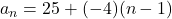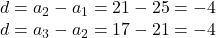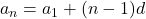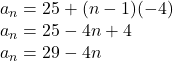## Question 1 1 pts Given the sequence: 25, 21, 17, 13,… Write the explicit equation that models the sequence.

Question

Question 1
1 pts
Given the sequence:
25, 21, 17, 13,…
Write the explicit equation that models the sequence.
A. an
4n + 29
B. an
25 +(-4)(n-1)
C. an = -25 +(-4)(n-1)
D. an
= 4 + 25
Edit
View
Insert
Format
Tools
Table

in progress 0
4 months 2021-09-04T17:09:53+00:00 1 Answers 242 views 0

The correct answer is: Option B:Step-by-step explanation:

Given sequence is:

25, 21, 17, 13,…

Here

a1 = 25

a2 = 21

a3 = 17

First of all we have to determine the common difference. Common difference is the difference between consecutive terms of an arithmetic sequence.

So,The explicit formula is given by:Putting the value of a1 and dHence,

The correct answer is: Option B: